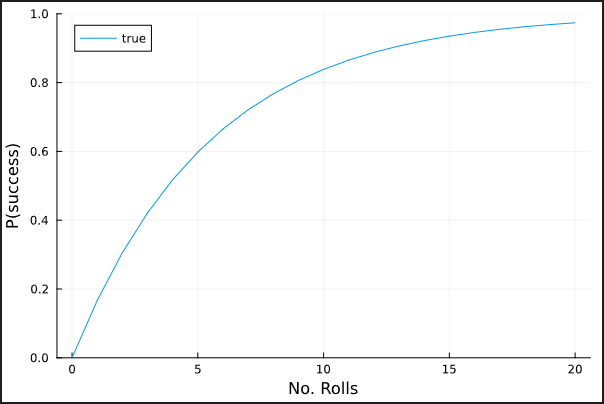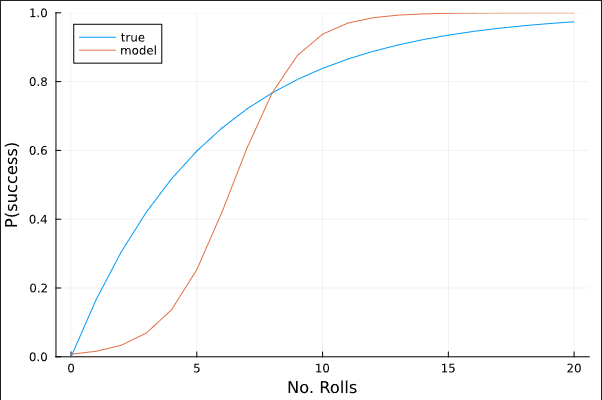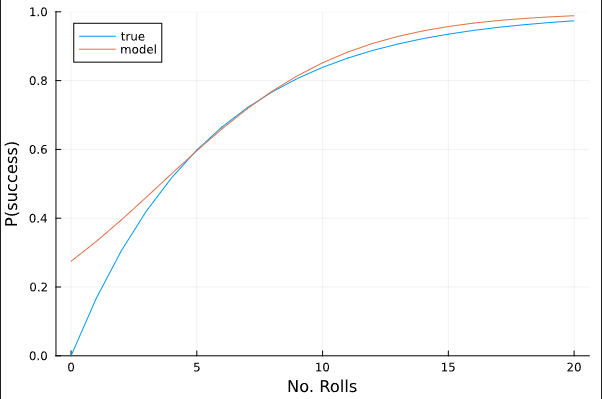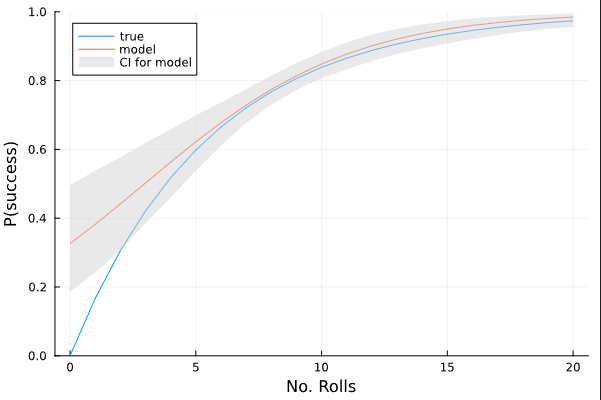Assume the following: I repeat a game over and over in which I roll a die a number of times. If I roll a 6 on any of the trials, I win. Otherwise, I lose. I want to model how the probability of winning this game changes as the number of trials increases.

In this case, I obviously know the answer when I’m using a standard 6-sided die:

``````using StatsPlots
# probability of rolling a six on a single throw
p = 1/6
# probability as a function of N (number of rolls)
P(N) = 1 - (1 - p)^N

plot(
P,
0:20;
ylims=(0,1),
label="true",
xlabel="No. Rolls",
ylabel="P(success)"
)
``````Let’s assume, though, that I do not know the probability of success for a given trial and I do not know how this probability is related to the number of trials. I just have data that show how many trials I ran during each round, and whether or not I won:

``````using DataFrames
using Distributions

data = DataFrame(num_rolls = rand(5:14, 300))
data.success = [rand(Binomial(row.num_rolls, p)) > 0 for row in eachrow(data)]

300×2 DataFrame
Row │ num_rolls  success
│ Int64      Bool
─────┼────────────────────
1 │        10     true
2 │        12    false
3 │        11     true
4 │        14     true
5 │        10     true
⋮  │     ⋮         ⋮
297 │         7     true
298 │         5     true
299 │         7    false
300 │         5     true
291 rows omitted
``````

An important note here is that I’ve deliberately chosen N to be a random number between 5 and 14, since that results in an average success rate of roughly 80%, most of the time.

Since the variable of interest is binary, logistic regression is a common choice. I implement it as follows:

``````using StatsFuns
using Turing

@model function success_model(success, num_rolls)
α ~ Normal(-5, 0.1)
β ~ Normal(0, 1.5)
for i ∈ eachindex(success)
p = logistic(α + β * num_rolls[i])
success[i] ~ Bernoulli(p)
end
end
``````

I’ve used a really strong prior for my intercept term since I know that the probability of success is zero when the number of rolls is zero. Now I fit my model and compare its average predictions across different values of N to the actual values:

``````m = success_model(data.success, data.num_rolls)
chain = sample(m, NUTS(), 1_000)
chaindf = DataFrame(chain)
ᾱ = mean(chaindf.α)
β̄ = mean(chaindf.β)
plot(
P,
0:20;
ylims=(0,1),
label="true",
xlabel="No. Rolls",
ylabel="P(success)"
)

plot!(
x -> logistic(ᾱ + β̄ * x),
0:20,
label="model"
)
``````As you can see, this is a pretty terrible model. My next thought was to get rid of the strong prior on the intercept term, so I did that by simply increasing the standard deviation to 2:

``````@model function success_model(success, num_rolls)
α ~ Normal(-5, 2)
β ~ Normal(0, 1.5)
for i ∈ eachindex(success)
p = logistic(α + β * num_rolls[i])
success[i] ~ Bernoulli(p)
end
end
``````

Now, I have this:This is a lot better, but still not very satisfying. This model is telling me I have a nonsensical 25% chance (roughly) of success if I don’t roll the die a single time. I’ve played around with different priors for the intercept term and there seems to be this tradeoff between having the model be grounded in reality when N = 0, and having it produce accurate results for higher values of N. I also tried dropping the intercept term completely, and that wasn’t good either. In addition to that, I tried balancing the data by oversampling the values where `success == false`, again to no avail.

I guess my question is: is there a better link function I can use that will give a more accurate representation of my true underlying model? Or, is it simply a matter of being aware that predictions are less accurate for lower values of N? I can visualize a confidence interval for the average probability of success across different values of N and see that the model has less confidence at lower values of N:

``````μ = [
logistic(row.α + row.β * x)
for row in eachrow(chaindf), x in 0:20
]
μ̄ = [mean(c) for c in eachcol(μ)]
μ₉₀ = hcat([percentile(c, [5,95]) for c in eachcol(μ)]...)'

plot!(
0:20,
[μ̄ μ̄],
fillrange=μ₉₀,
labels=["CI for model" ""],
color=:lightgrey,
alpha=0.5,
linewidth=0,
)
``````Or, lastly, is it just a matter of choosing wisely the threshold value that is used when using the model for prediction tasks? I would love to hear thoughts/advice/feedback on how to model this kind of problem. I have a real-world problem that is loosely analogous to this example and it’s pretty scary knowing I can get such dramatically different results, depending on my choice of a prior. I thought I was doing the right thing with the strong prior for the intercept, since I know for sure that there is no chance of success when N = 0, but I see in this example that doing so results in a really terrible fit.

You could try a variation of this function (a simplified version of the Type II functional response from ecology, or the Beverton-Holt stock-recruitment curve from fisheries science):

``````link(x, a) = x / (a + x)
``````

It always goes through the origin, and asymptotes to 1, with the slope at the origin given by `1/a`.

1 Like

Actually, I guess this isn’t exactly a “link” function in the usual GLM sense.

It is interesting to think about how you’d approach a simple problem like this without knowing the true data-generating process. I think most GLM modelers would see a bad fit and start adding quadratic (and maybe higher) terms to their linear predictor.

1 Like

I added a quadratic term and, with the right choice of standard deviation in the prior, it does result in a better fit. However, it is very sensitive to the choice of standard deviation, so it didn’t seem like much better an option for my real problem. I’ll play around with your `link` function and see what happens1-exp(-pred) would be my first choice probably.

1 Like

This problem actually reminded me of this great blog post from a few years ago about modeling the probability of sinking a golf putt as a function of distance from the hole:

https://mc-stan.org/users/documentation/case-studies/golf.html

1 Like